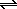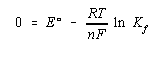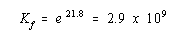Practice Problem 11

Use the following standard-state cell potentials to calculate the complex formation equilibrium constant for the Zn(NH3)42+ complex ion.

 Zn(NH3)42+ + 2 e-Zn + 4 NH3 Eored = -1.04 V Zn2+ + 2 e-Zn Eored = -0.7628 V

Solution

By reversing the first half-reaction and adding it to the second, we can obtain an overall equation that corresponds to the complex formation equilibrium.

 Zn + 4 NH3Zn(NH3)42+ + 2 e- Eoox = 1.04 V + Zn2+ + 2 e-Zn Eored = -0.7628 V ¯¯¯¯¯¯¯¯¯¯¯¯¯¯¯¯¯¯¯¯¯¯¯ ¯¯¯¯¯¯¯¯¯¯¯¯¯¯¯¯¯ Zn2+ + 4 NH3Zn(NH3)42+ Eo = 0.28 V

When this reaction comes to equilibrium, the cell potential is zero and the reaction quotient is equal to the complex formation equilibrium constant for the Zn(NH3)42+ complex ion.We now solve this equation for the natural logarithm of the equilibrium constant and calculate the value of Kf for this reaction.